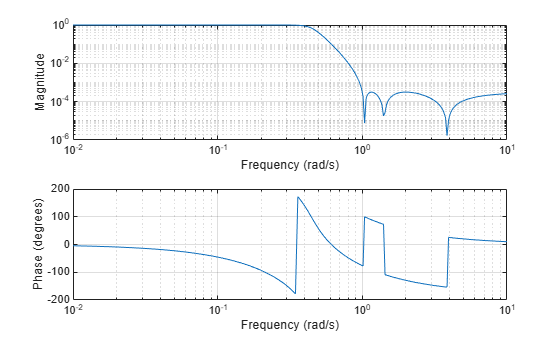# cheb2ap

Chebyshev Type II analog lowpass filter prototype

## Syntax

``[z,p,k] = cheb2ap(n,Rs)``

## Description

example

````[z,p,k] = cheb2ap(n,Rs)` returns the zeros, poles, and gain of an order `n` Chebyshev Type II analog lowpass filter prototype with `Rs` dB of ripple down from the passband peak value in the stopband.```

## Examples

collapse all

Design a 6th-order Chebyshev Type II analog lowpass filter with 70 dB of ripple in the stopband. Display its magnitude and phase responses.

```[z,p,k] = cheb2ap(6,70); % Lowpass filter prototype [num,den] = zp2tf(z,p,k); % Convert to transfer function form freqs(num,den) % Frequency response of analog filter```## Input Arguments

collapse all

Filter order, specified as an integer.

Data Types: `single` | `double`

Stopband ripple, specified as a scalar in decibels.

Data Types: `single` | `double`

## Output Arguments

collapse all

Zeros of the filter, returned as an `n`-length column vector. If `n` is odd, `z` has length `n`–1.

Poles of the filter, returned as an `n`-length column vector.

Gain of the filter, returned as a scalar.

## Algorithms

Chebyshev Type II filters are monotonic in the passband and equiripple in the stopband. The pole locations are the inverse of the pole locations of the `cheb1ap` function, whose poles are evenly spaced about an ellipse in the left half plane. The Chebyshev Type II stopband edge angular frequency ω0 is set to 1 for a normalized result. This value is the frequency at which the stopband begins. The filter has a magnitude response of 10–Rs/20.

Chebyshev Type II filters are sometimes called inverse Chebyshev filters because of their relationship to Chebyshev Type I filters. The `cheb2ap` function is a modification of the Chebyshev Type I prototype algorithm:

1. `cheb2ap` replaces the frequency variable ω with 1/ω, turning the lowpass filter into a highpass filter while preserving the performance at ω = 1.

2. `cheb2ap` subtracts the filter transfer function from unity.

The transfer function is given by

`$H\left(s\right)=\frac{z\left(s\right)}{p\left(s\right)}=k\frac{\left(s-z\left(1\right)\right)\left(s-z\left(2\right)\right)...\left(s-z\left(n\right)\right)}{\left(s-p\left(1\right)\right)\left(s-p\left(2\right)\right)...\left(s-p\left(n\right)\right)}.$`

 Parks, Thomas W., and C. Sidney Burrus. Digital Filter Design. New York: John Wiley & Sons, 1987.